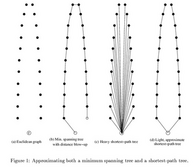## neal young / Khuller95Balancing

• Algorithmica 14(4):305-322(1995); SODA'93This paper has a simple linear-time algorithm to find a spanning tree that simultaneously approximates a shortest-path tree and a minimum spanning tree. The algorithm provides a continuous trade-off: given the two trees and $$\epsilon > 0$$, the algorithm returns a spanning tree in which the distance between any vertex and the root of the shortest-path tree is at most $$1+\epsilon$$ times the shortest-path distance, and yet the total weight of the tree is at most $$1+2/\epsilon$$ times the weight of a minimum spanning tree. This is the best trade-off possible.
Journal version of .